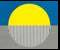COMPRESSIBLE FLOW Part 1: Energy, Relativity, Radiation Release

• Introduction
• How Much Energy is Required to Explain the Image Formation on the Shroud?
• The Nature of Energy
• Compressible Energy Flow:  A Basic Variable Speed of Light (VSL) Theory
• Compressible Energy Flow and Relativity
• Release of Radiation in a World A→ World B Transformation of Matter
1. The hidden mass or ‘dark’  matter of the Universe:  A New Solution
2. Release of radiation in an A to B transformation of matter and the problem of the Shroud’s image formation
• ReferencesINTRODUCTION

It is now necessary to turn to physics in the continuing investigation of the mystery of how the image on the Holy Shroud of Turin originated.  This is because it is scientifically well established that the image resides in a chemical alteration of the linen fibrils of the Shroud, and that this chemical alteration was probably caused by some form of radiation. But, radiation necessarily involves energy, and so we must investigate the amount of energy needed to form the image, and then its nature and source. As we shall see, a new theory of the compressibility of energy flows offers a promising solution to the source of the image forming radiation.

HOW MUCH ENERGY IS NEEDED TO EXPLAIN THE IMAGE FORMATION ON THE SHROUD?

A fundamental requirement of any consistent theory of image formation will have to be a consistent energy balance. The minimum energy required to form an image on the Shroud can be estimated as follows:  First, it is known that the image, although not a scorch, is similar to that which can be produced on linen by a slight thermal scorch [Pellicori, 1].  And so the chemical energy for any process which produces a similar image should be roughly equal to the scorch energy, which is readily calculable [Power, 2]. Eventually, of course, the precise (non-scorch) process of image formation needs to be established, but one can at least establish a preliminary general level of the energy needed to form the image by calculating the energy as though it were a scorch process.

The energy needed to scorch a given weight of linen can be calculated from the following heat equation:

Q = m ΔT cp                                                                                                                                       (1)

Where, m is the mass of linen scorched, ΔT is the temperature rise required to reach the scorch temperature of linen which is around 200ºC ( i.e. ΔT = 200 - 15 = 185ºC), cp is the specific heat of cellulose ( 0.34 calories per gram per degree C temperature rise; when Q the heat energy is expressed in Joules this is 0.34 x 4.186 = 1.423 Joules of heat energy per gram of linen scorched per degree temperature rise).

The mass m of linen scorched must be estimated. We know that the image is superficial and resides only on the crowns of the linen threads and moreover only on the outermost fibrils in each thread. There are from 80 to 100 fibrils in each thread. We therefore estimate that the mass of linen scorched lies roughly in the range of 1 to 50 grams.  Based on this mass estimate we can calculate the heat energy Q needed to chemically discolor the cellulose of the linen and form the image as follows:

 Estimated Mass (m) of Image Fibrils Scorched       (grams) Heat Energy (Q)  for Scorching (Joules) 1 286 10 2.86x103 20 5.72x103 40 1.14x104 50 1.43x104

The first thing to note is that these energies for image formation are actually small. Consequently, our image process can be quite tranquil or weak. It certainly need not be violent.  Nuclear or atomic disintegrations, which have sometimes been suggested as being involved, can now be ruled out on energy considerations, as well on the obvious physical integrity of the linen itself, since it bears no signs of any violent image formation process.

It must be emphasized that these energy estimates represent only the minimum energies needed to form the image by bringing the temperature of the linen up from room temperature ( 15ºC) to scorch temperature of 200ºC. This is because one can envisage that the energy emitted in some unknown process might exceed this minimum amount required for a scorch, but that the excess might then simply pass through the linen without further affecting it.  So, with this in mind, we can interpret the heat energy estimates as the minimum amounts needed and  not the actual amounts that might be, say, emitted, by the Body of the Man in the Shroud, in some physical transformation to be investigated later.

We conclude then that the minimum energy involved in forming the image on the Shroud probably lies in the range of 286 to 10,000 joules.

THE NATURE OF ENERGY

The name energy is derived from the Greek  energeia, meaning ‘relating to work’ or ‘relating to activity’ [ Gk. energes  from en-on + ergon- work].

The precise scientific concept of energy in science emerged only quite late in the 19th century. Energy is still today a somewhat subtle concept. This is probably due to the fact that it emerges from the mechanics of motion, not as a directly observable entity, but only as a calculated quantity [½ mV2] which is conserved or unchanged in magnitude during physical motions (V).  The concept of energy is so basic that the law of conservation of energy is probably the most fundamental law of science.

The kind of energy associated with the movement of material bodies is called kinetic energy [1/2mV2].

A second kind or category of energy is potential energy (U) .  This is stored energy, as in a coiled spring, or an energy of position, such as the stored gravitational energy of a body elevated above the earth’s surface. Potential or stored energy may be released and become kinetic energy of motion (1/2mV2), for example, if a suspended body is released and allowed to fall.

In wave motion, if c is the speed of the wave, then (m) c2 is its kinetic wave energy, with unit mass assumed so that m does not appear specifically in the equation. In a complete treatment of the subject, other kinds of energy such as electrostatic and electromagnetic energies, gravitational energy, chemical energy, surface free energy and so on, must be included, but in our present approach the mechanical energy will suffice.

Since energy is always conserved in any physical change, it is obviously a very powerful scientific concept. As so it is a curious fact that mechanics can actually be formulated (in terms of Newtonian force and momentum) without ever bringing the concept of energy in at all.  However, alternatively, it is also possible to formulate mechanics entirely from the concept of energy, and this approach, the Hamiltonian formulation, results in the most general and powerful formulation of the science of mechanics. It is little wonder that energy appears as a fundamental but still subtle concept [Lindsay and Margenau, 3].

This has led the philosopher Lonergan  to ask whether energy may be related to the philosophical concept of prime potency, the ancient and enduring philosophical principle proposed by Aristotle and other Greek philosophers as being the underlying entity constituting or ‘informing’ all physical matter, but which never appears or exists by itself or per se.

Clearly, energy is a very basic concept, and its conservative property permits us to approach the problem of the image formation on the Shroud on a fundamental and quantitative basis.

COMPRESSIBLE ENERGY FLOW AND THE IMAGE FORMATION ON THE HOLY SHROUD; Variable

Speed of Light (VSL) Theories

Since the amount of radiation energy that we have calculated above as needed to form the observed image on the Shroud is so small, we obviously need a physical mechanism to account for this. As we shall show later, the mechanism of  compressible energy flows gives us a concrete, testable explanation for the weak radiant energy release required to form the image observed on the Holy Shroud of Turin.

Compressible energy flow theory, is an application of well established, standard gas dynamics, aerodynamics, and atmospheric science theory [5,6], and  is therefore based on a century of  scientific and engineering results.

In addition, because the compressible energy flow equation contains a parameter (n) which represents the number of ways the energy of the system is divided, and which can have either positive or negative integral values, the theory introduces the possibility of a binary universe in which transformations of matter can take place involving release of radiant energy. This is the key to its potential ability to account for a release of the weak radiation from the dead body of The Man in the Shroud needed to form the image on the linen cloth.

Since compressibility of energy flow involves changes or transformations in physical matter such as atoms and electrons, it also  has cosmological and astronomical implications which may go far towards solving some of the central and most vexing scientific problems in physics, astrophysics and cosmology today, since it offers unique explanations for the so-called dark matter and dark energy of the universe, the mysterious physical entities which are known to constitute 96% of the physical universe, but as to whose physical nature, standard models of physics at the present time  have no explanation. It also provides a physically-based explanation for a hypothetical, enormous initial expansion of the cosmos called inflation which has been postulated in order to explain the observed uniformity of the universe.

Several recent approaches towards solving these major problems in physics and astrophysics have involved  an abandonment of the current fixed value for the speed of light c in space ( 3 x 108 m/s) required by Einstein’s theory of relativity.  Such theories are called Variable Speed of Light Theories (VSL). Currently, most of these proposals are still in the stage of ad hoc speculation (Moffat, Magueijo, Barrow, Youm,7,8,9,10).

But compressible energy flow is different (Power, 11,12,13,14,15,16,17) since it  introduces a variable speed of light as a physically based requirement from the outset, and moreover the variability in light speed is already experimentally verified, as we shall see. The standard, steady flow, compressible energy equation is [2,3]:

Ec = c2 = co2 - V2/n                                                                                (1)

or, expressed in words: (wave energy c2 at  any moment ) = ( maximum wave energy  co2  before any  flow) minus ( a portion of the kinetic energy of   flow,  V2/n)

Note: We should point out here that in compressible flow theory the mass m involved is assumed to be unity (m = 1) so that the mass doesn’t need to appear explicitly  in the equations.  If the mass were to be made explicit then the compressible energy equation would be written as

E c = (m)c2 = (m)co2 – (m)V2/n

In the equation, c is the variable compressive wave speed in a fluid , co is the usual constant, or  static wave speed of the fluid in the absence of any relative flow V, and n has values 1,2,3….. Here n specifies the number of ways the energy of the compressible flow is divided. In a material gas, such as air, for example, n is equal to 5 (three space motions for the air molecules in the x, y, and z directions, plus one each for the molecular rotation and vibration motions, making a total of 5). In air the static wave speed co, is the speed of sound, which at sea level is about 330 m/s ( 1080 feet per second).

The compressible energy flow equation may of course also be expressed in graphical form.  If the energies are plotted directly , that is as  c2  and   V2 ,  we have a set of straight line graphs of different slopes depending  on which value for n is assigned ( n = 1,2,3,4, etc.).   If the velocities V and c are plotted instead, we have either an elliptical set of curves for positive n or a hyperbolic set of curves for negative values of n.

c2 = co2 - V2/n    ( ±n)In standard meteorological and aeronautical practice n is usually taken as having the value 5 (i.e. the value of n for air is 5). But in general, in other fluid flow cases, n can have a wide range of values, and moreover it can be either positive or negative, and so this gives rise to two theoretical physical states. Hence, the possibility also arises that our universe is Binary.

The rather extraordinary cosmological properties of the two families of solutions of the compressible energy equation will be explored later in this discussion. As pointed out above, the transformation from World A matter to World B matter, if it were to take place, would involve the  release of small amounts of energy, and such a transformation of matter  is a prime candidate for the source of the small amount of radiation which could have formed the image on the Shroud.

COMPRESSIBLE ENERGY FLOW AND RELATIVITY

The compressible flow velocity V in Equation 1 is specified as being relative flow. One might suspect therefore that compressible flow has something to say about the famous theory of relativity, and this is indeed so.  For example, if we were to show the mass m explicitly in Eqn. 1, we would have

(m)c2 = (m)co2 - (m) V2/n

or

E = (m)c2 = (m)co2 – (m) V2/n

which has the form of the familiar special relativity mass/energy relationship (E = mc2)  but  now with a variable wave speed c and the addition of a  kinetic energy term  mV2 , and all  now  derived on purely physical grounds.

This problem with light arose very soon after the nature of light as an electromagnetic wave was discovered in the late nineteenth century. The addition of the speed of light c to  the velocity V of a light measuring instrument, for example, an interferometer mounted on the earth which is moving through space at V = 30 km/s around the sun, was soon found to be a big problem. Compressible flow was then not at all well understood, and so the addition of velocities was attempted using only the classical or so-called ‘Galilean’ method which is the direct addition and subtraction of V and c [  i.e. V +c) and (V- c)].

How this attempt failed, and how the solution embodied in  Einstein’s special relativity theory came to gradually be adopted, and the revisions to it now indicated as necessary by the new compressible energy flow approach are too detailed to present here.  Instead a new Website has been opened for a detailed scientific presentation of the theory at ( www.energycompressibility.info ). See also Note 18. below.

In any case, our main aim here is applying the new compressible energy theory to the problem of how radiation may have formed the image on the Shroud.

RELEASE OF RADIATION IN WORLD A → WORLD B TRANSFORMATIONS OF MATTER

Returning now to the problem of the nature and source of the weak radiation which could have formed the observed image on the Shroud, we note that in the basic compressible energy flow equation the parameter n ( which gives  the number of different ways the energy of the system is split up)  can have two values,  either positive or negative, for example  + 1 or -1, + 9 or -9, and so on.

.

Each value then describes an entirely different form of matter

2 = co2 - V2/n    ( ±n)1. The Hidden Mass or ‘Dark Matter’ of the Universe: a New Solution

Currently, the observed astronomical motions of certain structures of the universe, such as spiral galaxies, can be explained by Newton’s laws of motion only with the assumption that there is present in and around the structures additional ‘hidden’, optically and electromagnetically unobservable, ‘ dark matter’ amounting to from 90% to as much as 99% of the total mass.   The optically and electromagnetically observed mass, therefore, constitutes only from 10% to as little as 1% of the total mass required to explain the motions.  The existence and nature of this dark matter is a central problem of cosmology today.

In all known material gases n is a positive integer, but the possibility of it being alternatively a negative number, either integral or fractional,  raises the possibility of a binary, evolving universe encompassing both our ordinary, condensed energy matter and the astronomical ‘dark matter’.

The evolution of our cosmos might then be considered as (1) a World A with all +n matter,  (2) gradually transforming into a binary world of mixed  A  (+n) matter and B ( -n)  matter ( i.e. our ordinary optically observable  matter plus some rarefied negative n or  ‘dark matter’)  all evolving towards (3) a final  Unitary World B in which all matter has been transformed into rarefied or celeston matter.2. Radiation released in an A to B transformation and the problem of the Shroud’s image formation

The energy change ΔE in the transformation A → B is given from the energy equations

ΔE = cB2 - cA2 = V2 [1/nA - 1/nB]

Since nA is positive and nB is negative, energy must be evolved in the A → B transformations.

This is our proposed source of the relatively weak energy needed to form the Shroud image. It will be further explored in the future updates to to this page of the Website.

REFERENCES

1. S.F. Pellicori,  Spectral Properties of the Shroud of Turin, J. Applied Optics. 19, 12, p 1913, June 15, 1980.

2. Power, Bernard A., Shock Waves in a Photon Gas. Contr. Paper No. 203, American Association for the Advancement of Science, Ann. Meeting, Toronto, Jan. 1981.

-----------------NASA Requested Proposal: Control No. K- 2453;  Date:, 03-31-80. Implications of a Photon Shock Wave Effect for the Fizeau Experiment on the Velocity of Light in a Moving Medium.

3. R.B. Lindsay and H. Margenau,  Foundations of Physics. Dover reprint. Dover Publications Inc. New York. 1957.

4. Bernard J.F. Lonergan, Insight: A Study of Human Understanding. Philosophical Library, New York, 1957.

5. A. H. Shapiro, The Dynamics and Thermodynamics of Compressible Fluid Flow. 2 Vols. John Wiley and Sons, New York, 1953.

6.  R. Courant and K. O. Friedrichs,  Supersonic Flow and Shock Waves. Interscience, New York, 1948.

7. Joao Magueijo, Faster then the Speed of Light: The Story of a Scientific Speculation. Penguin Books, London. 2003. (In his book Magueijo credits John Moffat, of Toronto with a prior 1992 paper on VSL theory).

8.  John D. Barrow, and J. Magueijo,  Phys. Rev. Lett. B 443, 104-110, 1998.

9. John D. Barrow, Variations of alpha in space and time. Phys. Rev. D. 043515, 15Aug. 2002.

10. Donam Youm, Variable–speed-of-light cosmology and second law of thermodynamics. Physical Review D., 66, 043506, 15 Aug. 2002.

11. Power, Bernard A., Unification of Forces and Particle Production at an Oblique Radiation Shock Front. Contr. Paper N0. 462. American Association  for the Advancement of Science, Ann. Meeting,  Washington, D.C., Jan 1982.

12.  ----------------------, Baryon Mass-ratios and Degrees of Freedom in a Compressible Radiation Flow.  Contr. Paper No. 505. American Association for the Advancement of Science, Annual Meeting, Detroit, May 1983.

13. ------------------------ Il Meccanismo di Formazione dell’Immagine dela Sindon di Torino, Collegamento pro Sindone, Roma, Maggio-Giugno,  pp 13-28, 1997.

14. ------------------------, Caratterizzazione di una Lunghezza d’Onda per la Radiazione che Potrebe aver Creato I’Immagine Della Sindone di Torino. Collegamento pro Sindone, Roma. Novembre-Decembre, pp. 26-36, 1999.

15. -----------------------, An Unexpected Consequence of Radiation Theories of Image Formation for the Shroud of Turin. Proc. Worldwide Congress Sindone 2000,  Orvieto, Italy, Aug. 27-29, 2000.

16. -----------------------, Image Formation on the Holy Shroud of Turin by Attenuation of Radiation in Air.  Collegamento pro Sindone website (www.shroud.it/)   March 2002.

17. -----------------------, Summary of a Universal Physics, Dorval, Quebec, 1992. To be revised and reprinted in a future Update.

18. Compressible Flow and Special Relativity Compared:

1. Special Relativity ( Uniform Motion)

The theory requires a constant speed of light c and is restricted to uniform motions.

It is based on the physically contradictory proposition that the square of the speed of light c2  in a coordinate system which is not moving must be equivalent to c2 – V2.in another system which is moving at a uniform velocity V. That is to say, that

c2 –V2 = c2

Then, since the equation is algebraically unequal, it is restated with a correction parameter as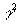(c2 – V2) = c2

Which, upon solving for the parameter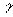, yields=  [1 – (V/co)2 ]-1/2 , the Fitzgerald/Lorentz/Einstein contraction factor which corrects for the contraction in the length of  moving bodes and for the time dilation of moving atomic clocks,.

The length contraction, given by l = l’, is not directly observable; it must be supported experimentally by  the absence of any fringe shift in Michelson-Morley  type experiments involving uniform relative motion V. In fact, however, small fringe shifts are always observed. Special relativity then rejects these observations declaring them to actually be zero.

An observable time dilation is predicted which is given experimentally in oscillator tests as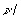o =  [1 – (V/co)2 ]-1/2.

The theory is compatible with the relation for mass and energy E = mc2 which, although the theory is restricted to uniform motion, must be verified by using highly accelerated mass particles.

2. Compressible Flow Yields a Universal Relativity (uniform and non-uniform motion)

The theory of compressible energy flow requires a variable speed of light c. It applies to both uniform and non-uniform motions. It therefore is a universal relativity.

It is based on the self- consistent physical theory of compressible fluid flow, which is verified by a century of experimental results for material fluids. It is here extended to energy flows involving the propagation of electromagnetic wave motions.

The basic equation is a physically verified energy equation

c2 = co2 –V2/n

where we now have a static maximum wave speed co when there is no relative motion V, an actual wave speed c which becomes smaller as the flow speed V increases, and an energy partition parameter n which designates the number of ways the energy of the system is divided.

The length contraction given by l = l’= l’[ 1  (1/n) (V/co2]-1/2  where= co/c, is predicted is  verified and observed via a fringe shift given by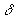= 2l (V/co)/n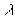, where n is the energy partition parameter. For Michelson- Morley type experiment with n = 9 the predicted  fringe shifts  are about one ninth of the classically predicted shifts  for an orbital motion V of 30 km’s, and these are always experimentally observed.

The time dilation is predicted  byo =  [1 –  (1/n)(V/co)2 ]-1/2 .This predicted time dilation is always  verified  in oscillator tests provided an appropriate value for n from 1 to 9 is used.

The mass energy relationship E = mc2 automatically arises from compressible theory when n = 1.

For unsteady motions, the relations in universal relativity become first order in the correction ratio (V/co). We have  c/co = 1/= 1- (1/n) (V/co) for length contraction ( fringe shift). For time dilation, we have  c/co =o =  1 –  (1/n)(V/co) via oscillator frequency change. For appropriate values of n these relations are verified by the observations..

While for material gases the energy partition parameter n can often be selected from theoretical knowledge of molecular motions (translation, rotation and vibration),  for electromagnetic applications its value in each case must at present  be determined from experiment. For Michelson-Morley type experiments n = 9,

For highly accelerated motions, n = 1 and the contraction factors of the two theories are then identical.

Conclusions

Special relativity is internally contradictory and is restricted to uniform motions. It predicts a length contraction which requires a zero fringe shift which is never observed so that it must reject the actual fringe shift observations. It predicts a time dilation which has to date only been verified in non-uniform motions. It is compatible with the mass/energy relation  E = m c2  and  this is verified only in strongly non-uniform motions.

Compressible flow relativity is physically based and self-consistent . It yields a universal relativity for both steady and unsteady motions. It accepts all the observations, both for length contraction and time dilation, and is verified by them.

Details on the full theory are available at www.energycompressibility.info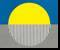Main Page